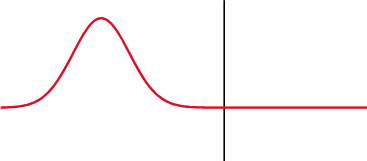# Transmission coefficient

Transmission coefficientright|frame|An electromagnetic (or any other) wave experiences partial transmittance and partial reflectance when the medium through which it travels suddenly changes.

The transmission coefficient is used in physics and electrical engineering when wave propagation in a medium containing discontinuities is considered. A transmission coefficient describes either the amplitude or the intensity of a transmitted wave relative to an incident wave.

Different fields have different definitions for the term.

Optics

In optics, transmission is the property of a substance to permit the passage of light, with some or none of the incident light being absorbed in the process. If some light is absorbed by the substance, then the transmitted light will be a combination of the wavelengths of the light that was transmitted and not absorbed. For example, a blue light filter appears blue because it absorbs red and green wavelengths. If white light is shone through the filter, the light transmitted also appears blue because of the absorption of the red and green wavelengths.

The transmission coefficient is a measure of how much of an electromagnetic wave (light) passes through a surface or an optical element. Transmission coefficients can be calculated for either the amplitude or the intensity of the wave. Either is calculated by taking the ratio of the value after the surface or element to the value before.

Quantum mechanics

In non-relativistic quantum mechanics, the transmission coefficient and related reflection coefficient are used to describe the behavior of waves incident on a barrier. The transmission coefficient represents the probability flux of the transmitted wave relative to that of the incident wave. It is often used to describe the probability of a particle tunneling through a barrier.

The transmission coefficient is defined in terms of the incident and transmitted probability current density j according to:::$T = frac\left\{|j_\left\{incident\right\},$ where jincident is the probability current in the wave incident upon the barrier and jtransmitted is the probability current in the wave moving away from the barrier on the other side.

The reflection coefficient R is defined analogously, as R=|jreflected|/|jincident|. Conservation of probability implies that T+R=1.

For sample calculations, see "finite potential barrier" or "square potential".

WKB approximation

Using the WKB approximation, one can obtain a tunnelling coefficient that looks like:$T = frac\left\{e^\left\{-2int_\left\{x_1\right\}^\left\{x_2\right\} dx sqrt\left\{frac\left\{2m\right\}\left\{hbar^2\right\} left\left( V\left(x\right) - E ight\right)\right\}\left\{ left\left( 1 + frac\left\{1\right\}\left\{4\right\} e^\left\{-2int_\left\{x_1\right\}^\left\{x_2\right\} dx sqrt\left\{frac\left\{2m\right\}\left\{hbar^2\right\} left\left( V\left(x\right) - E ight\right) ight\right)^2\right\}$

Where $x_1,x_2$ are the two classical turning points for the potential barrier. If we take the classical limit of all other physical parameters much larger than Planck's constant, abbreviated as $hbar ightarrow 0$, we see that the transmission coefficient correctly goes to zero. This classical limit would have failed in the situation of a square potential.

If the transmission coefficient is much less than 1, it can be approximated with the following formula:: $T approx 16 frac\left\{E\right\}\left\{U_0\right\} \left(1-frac\left\{E\right\}\left\{U_0\right\}\right) e^\left\{-2 L sqrt\left\{frac\left\{2m\right\}\left\{hbar^2\right\} \left(U_0-E\right)$where $L = x_2 - x_1$ is the length of the barrier potential.

Telecommunication

The transmission coefficient is the ratio of the amplitude of the complex transmitted wave to that of the incident wave at a discontinuity in the transmission line.

The probability that a portion of a communications system, such as a line, circuit, channel or trunk, will meet specified performance criteria is also sometimes called the "transmission coefficient" of that portion of the system. The value of the transmission coefficient is inversely related to the quality of the line, circuit, channel or trunk.

Chemistry

The transmission coefficient is a state of unity for monomolecular reactionshuh. It appears in the Arrhenius equationhuh.

References

* Telecommunication: Federal Standard 1037C in support of MIL-STD-188
*

Wikimedia Foundation. 2010.

### Look at other dictionaries:

• transmission coefficient — noun (physics) The probability of penetration of a nucleus by a particle striking it • • • Main Entry: ↑transmit …   Useful english dictionary

• transmission coefficient — perdavimo faktorius statusas T sritis Standartizacija ir metrologija apibrėžtis Dydis, lygus elektrinės grandinės išėjimo ir įėjimo signalų įtampų, srovių arba galių dalmeniui. atitikmenys: angl. transfer coefficient; transfer factor;… …   Penkiakalbis aiškinamasis metrologijos terminų žodynas

• transmission coefficient — transmisijos koeficientas statusas T sritis chemija apibrėžtis Aktyvinto komplekso dalelių dalis, skylanti į reakcijos produktus. atitikmenys: angl. transmission coefficient rus. трансмиссионный коэффициент …   Chemijos terminų aiškinamasis žodynas

• heat transmission coefficient — šilumos perdavimo koeficientas statusas T sritis fizika atitikmenys: angl. coefficient of heat transmission; heat transmission coefficient vok. Wärmedurchgangskoeffizient, m rus. коэффициент теплопередачи, m pranc. coefficient de transmission de… …   Fizikos terminų žodynas

• Heat Transmission Coefficient —   Any coefficient used to calculate heat transmission by conduction, convection, or radiation through materials or structures …   Energy terms

• transmission — [ trɑ̃smisjɔ̃ ] n. f. • XIVe, répandu XVIIIe XIXe; lat. transmissio I ♦ Fait, manière de transmettre, de se transmettre. 1 ♦ Action de transmettre (1o). Transmission d un bien, d un droit à une autre personne. ⇒ cession. La transmission des… …   Encyclopédie Universelle

• Transmission — is the act of passing something on.Specifically, it may refer to:*Transmission (mechanics), a gear system transmitting mechanical power, as in a car *Transmission (telecommunications), the act of transmitting messages over distances *Transmission …   Wikipedia

• Coefficient de transmission — ● Coefficient de transmission coefficient caractérisant le transfert de chaleur entre un solide (ou un fluide) à une certaine température et un solide (ou un fluide) à une autre température …   Encyclopédie Universelle

• coefficient de transmission de la chaleur — šilumos perdavimo koeficientas statusas T sritis fizika atitikmenys: angl. coefficient of heat transmission; heat transmission coefficient vok. Wärmedurchgangskoeffizient, m rus. коэффициент теплопередачи, m pranc. coefficient de transmission de… …   Fizikos terminų žodynas

• coefficient de transmission thermique — šilumos perdavimo koeficientas statusas T sritis fizika atitikmenys: angl. coefficient of heat transmission; heat transmission coefficient vok. Wärmedurchgangskoeffizient, m rus. коэффициент теплопередачи, m pranc. coefficient de transmission de… …   Fizikos terminų žodynas